Next: Facts Up: Basic Number Theory Previous: Axioms   Contents

## Definitions

•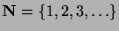and is called the set of natural numbers.
•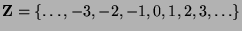and is called the set of integers.
•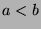means that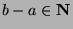.
•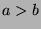means that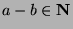.
•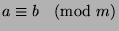means that a=b+mq for some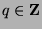.
•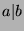means that there is an integer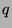such that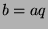.
•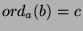means that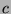has the following properties:
•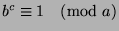.
•is the least such positive integer.
Note that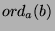exists if and only if (iff)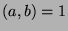.
•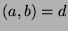means that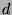has the following properties:
•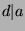.
•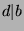.
• If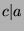and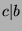and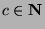then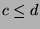.is called the greatest common divisor(GCD) of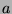and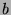.
•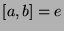means that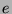has the following properties:
•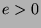.
•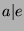.
•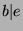.
• If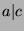and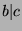andthen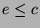.is called the least common multiple(LCM) ofand.

Gregory Stoll 2000-04-08